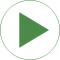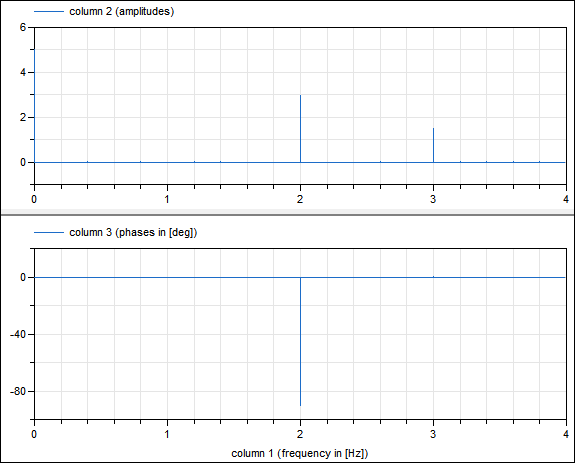# RealFFT1

Example demonstrating the use of an FFT calculation during simulation (and storing both amplitudes and phases on file)# Information

This information is part of the Modelica Standard Library maintained by the Modelica Association.

In this example the signal y

```   y = 5 + 3*sin(2*pi*f1) + 1.5*cos(2*pi*f2)
```

is sampled and an FFT is computed from the sampled signal (default: f1 = 2 Hz, f2 = 3 Hz). In the public part the FFT is stored up to f_max (internally in the protected part the FFT is stored up to 5*f_max). With the default values for f_max (= 4 Hz) and f_resolution (= 0.2 Hz), the following results are achieved:

```   fi  = 0,  Ai  = 5;   // mean value of signal
fi = 2,  Ai = 3;   // frequency/amplitude of sine
fi = 3,  Ai = 1.5; // frequency/amplitude of cosine
```

A plot of the resulting FFT is shown in the next image:Note, phases of small amplitudes (= smaller as 0.0001*maximalAmplitude) are explicitly set to zero, since the corresponding "phase" is numerical noise (and would just be confusing). Furthermore, note that the FFT phases are with respect to a cos(..) signal.

# Parameters (7)

f_max Value: 4 Type: Frequency (Hz) Description: Maximum frequency of interest Value: 0.2 Type: Frequency (Hz) Description: Frequency resolution Value: 2 Type: Frequency (Hz) Description: Frequency of sine Value: 3 Type: Frequency (Hz) Description: Frequency of cosine Value: "RealFFT1_resultFFT.mat" Type: String Description: File where FFT will be stored as [f,A,Phi], with f in {Hz] and A the amplitudes and Phi the phases in [rad] Value: max(1, min(integer(ceil(f_max / f_resolution)) + 1, nf)) Type: Integer Description: Number of frequency points of the interested frequency range (only up to f_max) Value: Type: Frequency[nfi] (Hz) Description: FFT frequencies of interested frequency points

# Outputs (3)

Ai Type: Real[nfi] Description: FFT amplitudes of interested frequency points Type: Real[nfi] Description: FFT phases of interested frequency points Type: Integer Description: Information flag from FFT computation; = 0: FFT successfully computed